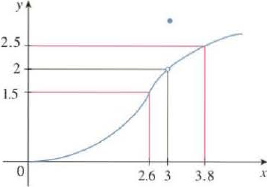Chapter 1.7, Problem 2E

Chapter
Section
Textbook Problem

Use the given graph of f to find a number δ such that i f   0 < | x − 3 | < δ   t h e n   | f ( x ) − 2 | < 0.5To determine

To find: The value of a number δ by using the graph of f.

Explanation

Given:

If 0<|x3|<δ, then |f(x)2|<0.5.

Definition used:

If for every number ε>0 there is a number δ>0 such that if 0<|xa|<δ, then |f(x)L|<ε.

Absolute value inequality: If |x|<a,a>0 then a<x<a.

Calculation:

Consider |f(x)2|<0.5.

By absolute value definition, 0.5<f(x)2<0.5.

Simplify the inequality by adding 2 on each side,

20.5<2+f(x)2<2+0

Still sussing out bartleby?

Check out a sample textbook solution.

See a sample solution

The Solution to Your Study Problems

Bartleby provides explanations to thousands of textbook problems written by our experts, many with advanced degrees!

Get Started

Evaluate the limit, if it exists. limx5x25x+6x5

Single Variable Calculus: Early Transcendentals, Volume I

In Exercises 19-24, find the functions f + g, f g, fg, and fg. 19. f(x) = x2 + 5; g(x) = x2

Applied Calculus for the Managerial, Life, and Social Sciences: A Brief Approach

Simplify: 1339

Elementary Technical Mathematics